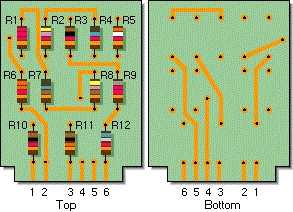# Electronics - Series Circuits

### Exercise :: Series Circuits - General Questions

21.If a voltage is connected between contacts 2 and 3 in the given circuit, which resistors will carry current?

 A. All twelve resistors will carry current. B. Only R6 and R11 will carry current. C. Only R2, R4, R6, and R11 will carry current. D. Only R3, R6, R9, and R12 will carry current.

Explanation:

No answer description available for this question. Let us discuss.

22.

Given a series circuit containing resistors of different values, which statement is not true?

 A. The current through each resistor is the same. B. The sum of the voltage drops across each resistive element will be equal to source voltage. C. The total resistance is the sum of the value of the resistors. D. The voltage drop across each resistor is the same.

Explanation:

No answer description available for this question. Let us discuss.

23.

With 20 V applied, an 8-ohm resistor is in series with a lamp. When the lamp is removed, what voltage will be read across the lamp socket?

 A. 0 V B. 8 V C. 12 V D. 20 V

Explanation:

No answer description available for this question. Let us discuss.

24.

When 50 V is applied to four series resistors, 100 µA flows. If R1 = 12 k, R2 = 47 k, and R3 = 57 k, what is the value of R4?

 A. 38.4 kB. 3.84 kC. 384 kD. 3.84 MExplanation:

No answer description available for this question. Let us discuss.

25.

In a series circuit, the voltage measured across a short will be:

 A. source voltage B. infinite voltage C. zero volts D. the normal voltage drop